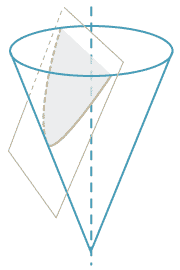## Motivation

Quadratic equations arose in antiquity in the context of problem solving. These problems were generally expressed verbally, but their solution involved some method for solving a quadratic equation — often also expressed verbally.Detailed description of diagram

The Greeks studied the parabola and its geometry. This curve occurs naturally as the edge of the cross-section of a cone sliced at an angle to its main axis.

The parabola can also be thought of as the graph of the quadratic function. It models the path taken by a ball thrown under gravity at an angle to the horizontal, assuming we ignore air resistance.

Since the Greeks did not have the notion of a coordinate system, their studies were heavily geometric and often hard to understand. With the advent of coordinate geometry, the study of the parabola became much easier and a full and complete analysis became possible.

In this module, we will summarise all of the main features and properties of quadratic equations and functions.

We will also look at a geometric definition of the parabola and its representation using parametric equations.

One of the many interesting characteristics of the parabola is its reflective property, used in collecting light from a distant source.

It is not intended that all the material in this module be taught in one topic in the classroom. Our aim here is to present the teacher with a comprehensive overview of quadratic functions and the geometry of the parabola.

Next page - Content - The basic parabola